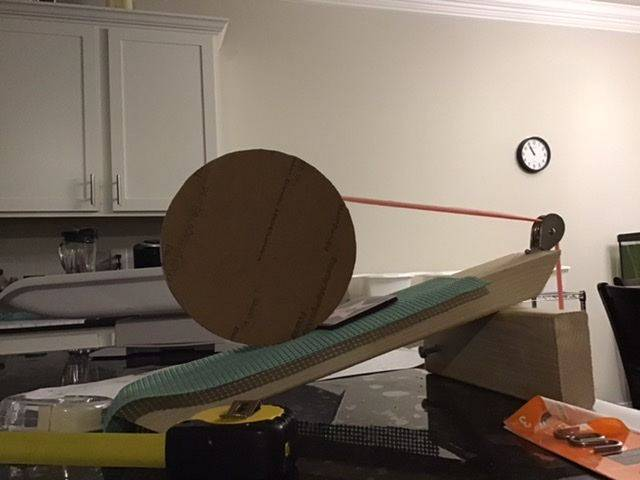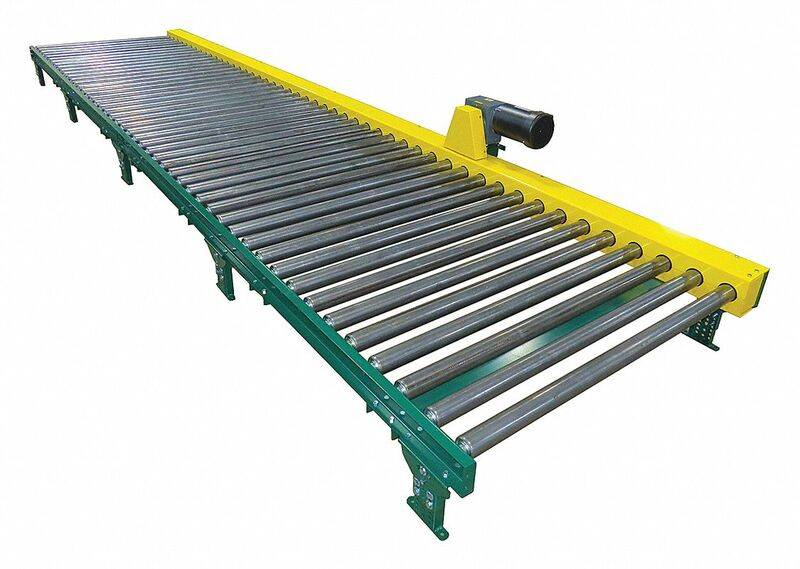# Can a static force do work?

• B
• leafy
Thanks guys. I did the experiment and measurements. And the result is conservation of energy win, again. Efficiency is around 40s%. I turn the wheel upside down and efficiency doesn’t change much. So yes, you guys are right. Sorry giving you a hard time Dale. I should have done this before discuss things. Oh well,Someday I’ll beat CoE.

••weirdoguy, Dale and Lnewqban
I did the experiment and measurements.
Excellent. That is science, sometimes it confirms your hypothesis and sometimes it doesn’t. But either way you learn something about the world.

••russ_watters, Lnewqban and ergospherical
If the wheel is moving at 1m/s, imparting a force while moving is equivalent of throwing at rock at greater than 1m/s and transfer energy. Matter of fact, let make the wheel move at 100m/s. You have to throw rock faster than that. Let's say the station pusher throw rock too to move the wheel, they only need to throw rock at approximately 0 m/s to impart the same force.
I'm not sure I entirely follow your scenario, but there is an important calculation relating to the acceleration of an object by throwing a projectile. Effectively rocket expellant. This came up recently, in fact.

First, we have a vehicle of large mass ##M## at rest on frictionless rails and we throw an object of small mass ##m## out the back at a speed ##u##, in the initial rest frame. By conservation of momentum, the speed of the vehicle Is $$\Delta v = \frac{m}{M}u$$which gives a gain in KE of $$\Delta KE = \frac 1 2 M(\Delta v)^2$$But, now we consider the same event in a reference frame where the vehicle has an initial speed of ##v_0##. Conservation of momentum gives the same value for ##\Delta v##, but the increase in KE of the vehicle is$$\Delta KE = \frac 1 2 M[(v_0 + \Delta v)^2 - v_0^2] = \frac 1 2 M[(\Delta v)^2 + 2v_0\Delta v]$$Which is dependent on ##v_0## and greater than before.

The question is then to figure out where the extra kinetic energy has come from?

imparting a force while moving cost a lot of energy
This is only true for certain systems. The term "a lot" is too wooly for serious discussion.

In general, it's possible to impart force for any speed. Just consider the use of bicycle gears. The high gear ratios allow the cyclist still to apply force (torque) to the wheel, at higher speed (else you cannot 'catch up with' the wheels). Of course, the Power delivered to the wheels goes down because
Power = Force times speed
but there is no cut off - you just get progressively less and less propulsive Force / torque as speed increases.

The problem with throwing example numbers at an explanation is that the pattern is easily missed. If you really want to get into the Physics then you need to express things with symbolic maths (algebra etc).

HAND that pulls the string is doing work. String by itself is like a gun with no one to pull the trigger.

Let's say I was pulling the string. I felt a tiny displacement. So my work done is the force I pull times the displacement my hand made. On the cylinder end, the same force carry by the string, but the displacement is many times higher. The work done is then force time big displacement. Obviously, the energy doesn't add up.
I think you must be careful about the 'displacement' as well. Work = f*d = m*a*d--but remember, it is ONLY work over the distance that the mass is <accelerating> and NOT the entire distance the mass moves. Apply a force of 1N to a 1 kg mass over a distance of 1 m on a frictionless surface and you will have done 1 J worth of work. But, being on a frictionless surface, that mass will move an infinite amount of distance over an infinite amount of time, since no other forces are acting on it to stop it.

Your spool will continue to roll up string after force is no longer applied to it until the forces of friction reduce its velocity to zero, but work stopped being added to the system when acceleration stopped. In your diagram in #10, work is only being done through small 'd.' If the system were frictionless, and the string infinitely long large 'D' would be infinite.

•weirdoguy
If the video in the OP makes your head spin, you'll trip while watching the following video (although this one gives some explanations):

•russ_watters
Thread is old, and I just skimmed, but did anyone say gearing/mechanical advantage? This is just a simple but clever gear train.

Work = f*d = m*a*d--but remember, it is ONLY work over the distance that the mass is <accelerating> and NOT the entire distance the mass moves.
W = F * d applies regardless whether that mass is accelerating. Here F is just some individual force, not the net force, so you cannot assume F = m * a. If the mass is moving at constant speed, an individual force F can still be doing work on it, while other forces do negative work, so it doesn't gain kinetic energy.

Let's say you're right that the motion of the material is important.

Easy way to see that @Dale is right: Consider a box on the powered rollers below. The location of the contact point between roller and box is not moving (in the rest frame of the machine), but the machine can obviously do work on the box and accelerate it. So it's the motion of the box material to which the force is applied to, that matters for work being done by that force on the box.Last edited:
•Dale
W = F * d applies regardless whether that mass is accelerating.
Overcoming frictional forces equates to accelerating an object (friction decelerates, you accelerate, net zero perhaps) In a frictionless environment, all force causes acceleration. The distance that matters is <not> the total distance that the object moves, but the distance involved in which the object's velocity is changing from what it would be if you did nothing. If you shove a 1 kg object on surface for 1 m and imparted a final velocity of 10 m/s to it, and then it took 99 m to return to rest, the work YOU did has nothing to do with the 99 m distance. The work that friction did in returning the object to rest has everything to do with the 99 m.

Agreed?

•weirdoguy
Overcoming frictional forces equates to accelerating an object (friction decelerates, you accelerate, net zero perhaps)
Frictional forces can act to increase or decrease speed, depending on the scenario and reference frame.

In a frictionless environment, all force causes acceleration.
No. You can have balanced non-frictional forces, and thus no acceleration.

The distance that matters is <not> the total distance that the object moves, but the distance involved in which the object's velocity is changing from what it would be if you did nothing.
None of that complicated what-would-happen-if-stuff is in the definition of work. The motion of the material to which the force is applied to, is what matters for work being done by that individual force.

•sophiecentaur, Dale and weirdoguy
The intuition with both spool and bike is very simple: if you pull on a string, it has to move toward you. (I remember seeing a claim that this wouldn't have to be true of tensegrity structures, but I have a hard time imagining that)

Whether the string spools or unspools when pulled is a case of inductive reasoning, but not "physics". There are no laws of physics that talk about how many times a string is wrapped around something. (Unless it's a cosmic string :) )